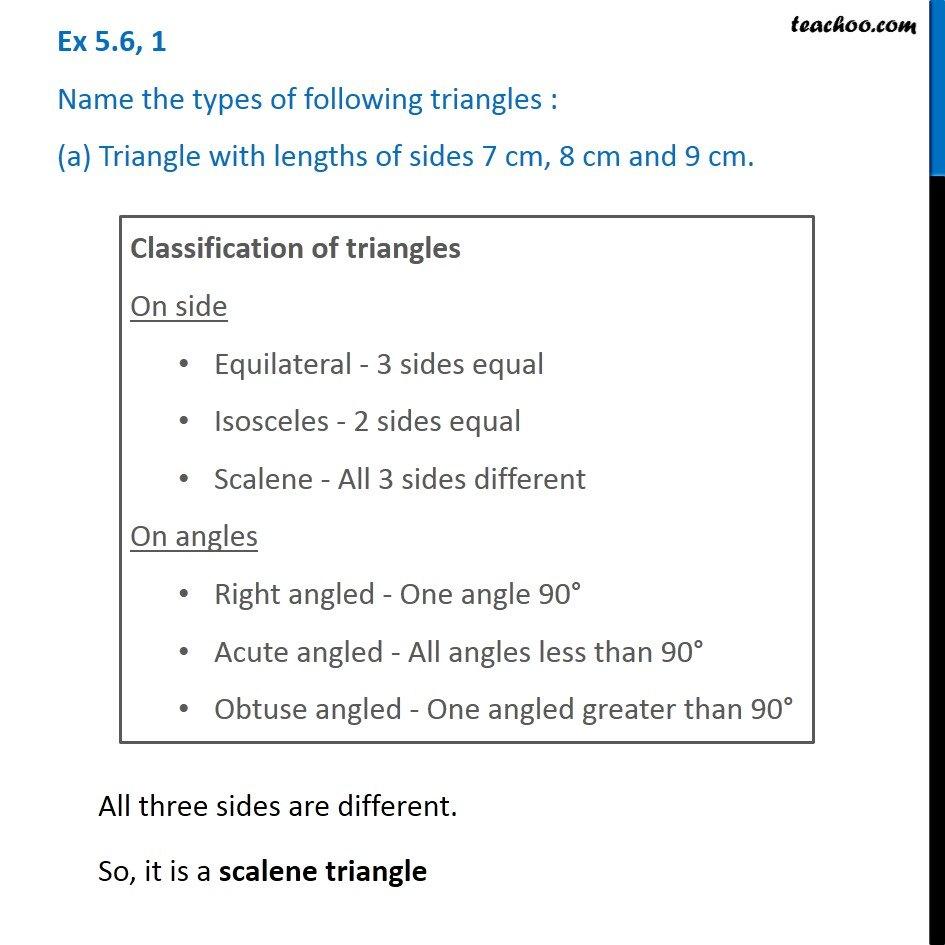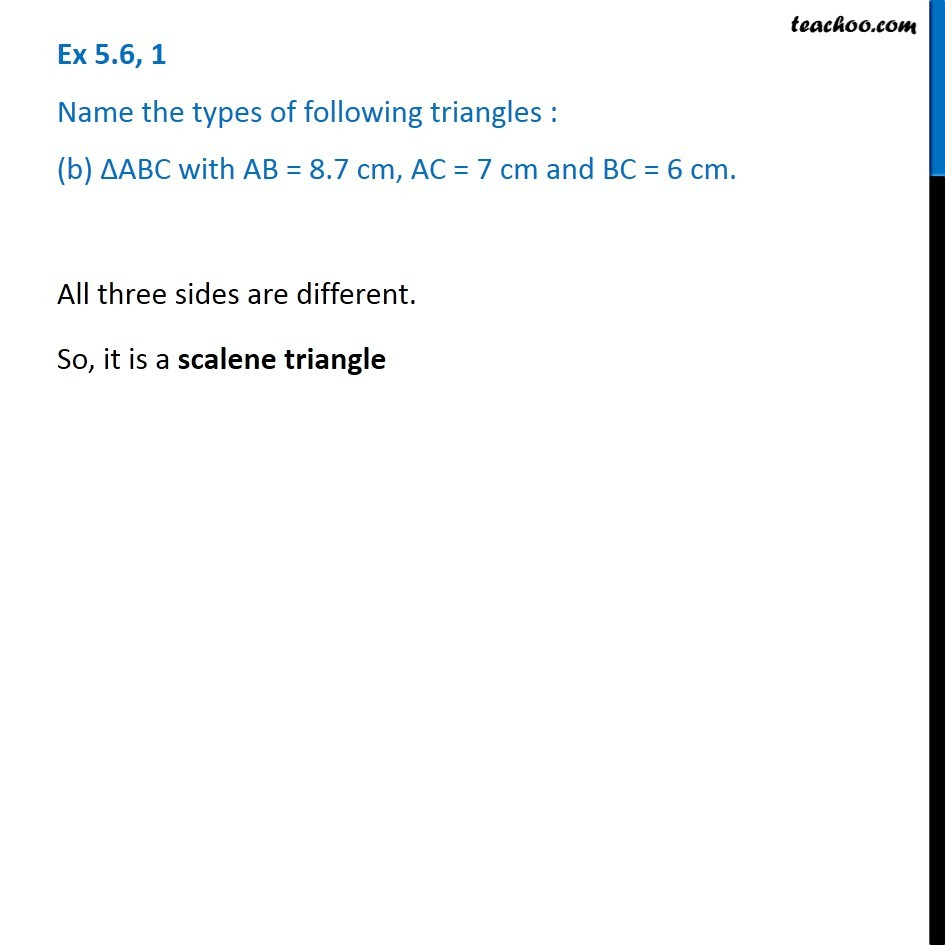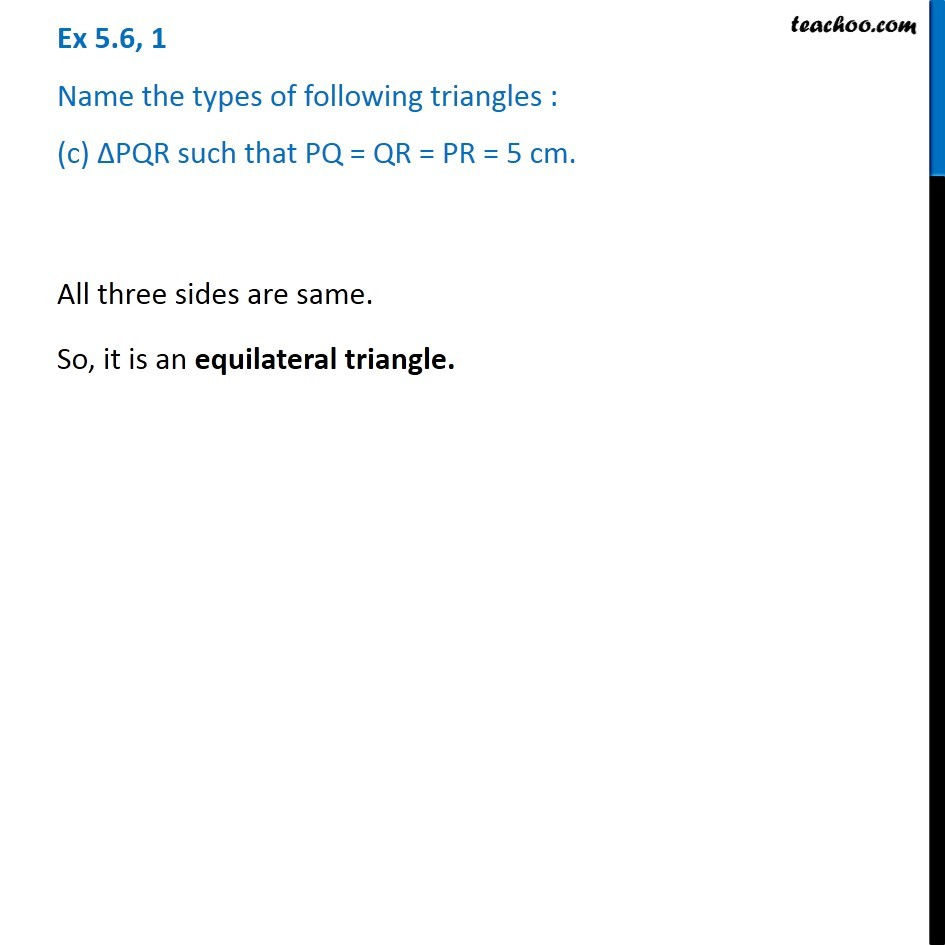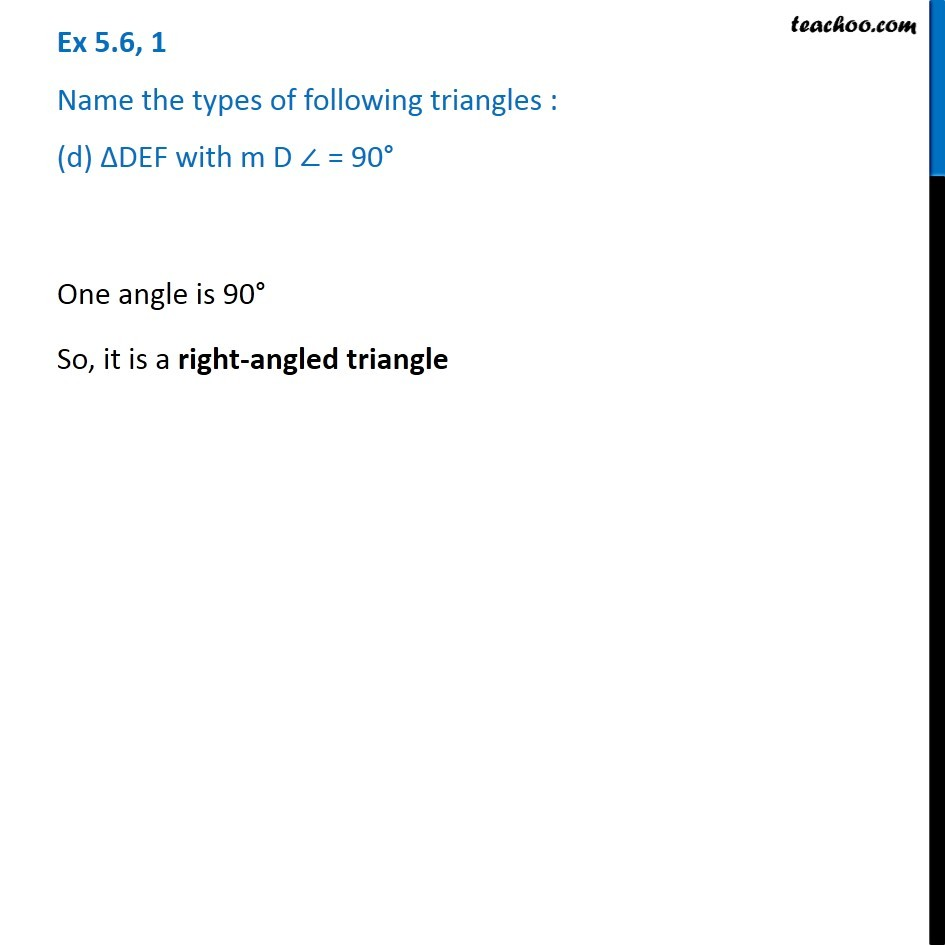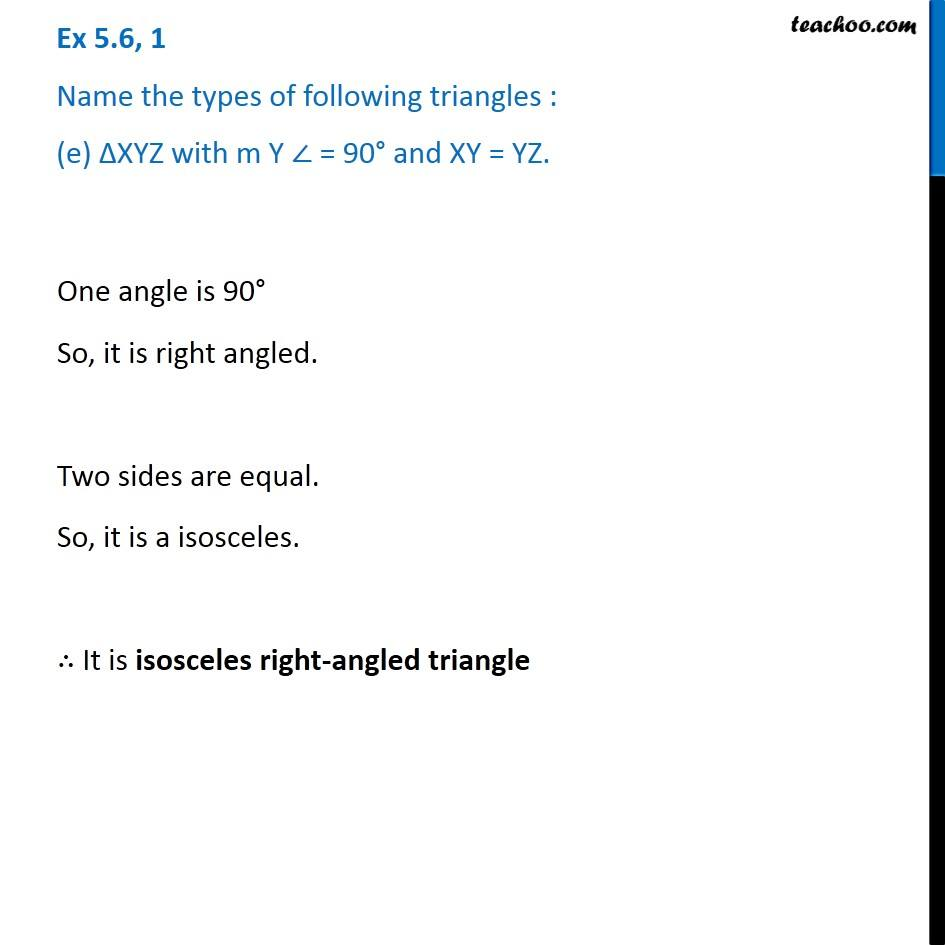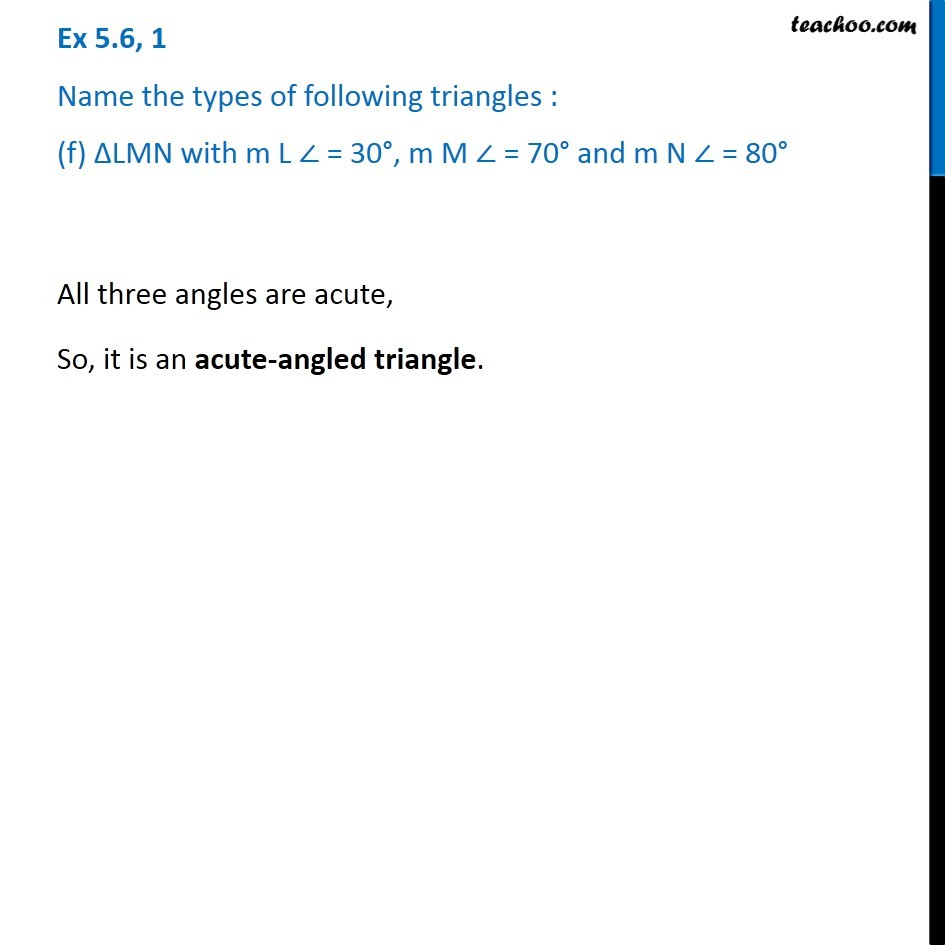Subscribe to our Youtube Channel - https://you.tube/teachoo

1. Chapter 5 Class 6 Understanding Elementary Shapes
2. Serial order wise
3. Ex 5.6

Transcript

Ex 5.6, 1 Name the types of following triangles : (a) Triangle with lengths of sides 7 cm, 8 cm and 9 cm. Classification of triangles On side Equilateral - 3 sides equal Isosceles - 2 sides equal Scalene - All 3 sides different On angles Right angled - One angle 90° Acute angled - All angles less than 90° Obtuse angled - One angled greater than 90° All three sides are different. So, it is a scalene triangle Ex 5.6, 1 Name the types of following triangles : (b) ∆ABC with AB = 8.7 cm, AC = 7 cm and BC = 6 cm. All three sides are different. So, it is a scalene triangle All three sides are different. So, it is a scalene triangle All three sides are same. So, it is an equilateral triangle. Ex 5.6, 1 Name the types of following triangles : (d) ∆DEF with m D ∠ = 90° One angle is 90° So, it is a right-angled triangle Ex 5.6, 1 Name the types of following triangles : (e) ∆XYZ with m Y ∠ = 90° and XY = YZ. One angle is 90° So, it is right angled. Two sides are equal. So, it is a isosceles. ∴ It is isosceles right-angled triangle Ex 5.6, 1 Name the types of following triangles : (f) ∆LMN with m L ∠ = 30°, m M ∠ = 70° and m N ∠ = 80° All three angles are acute, So, it is an acute-angled triangle.

Ex 5.6